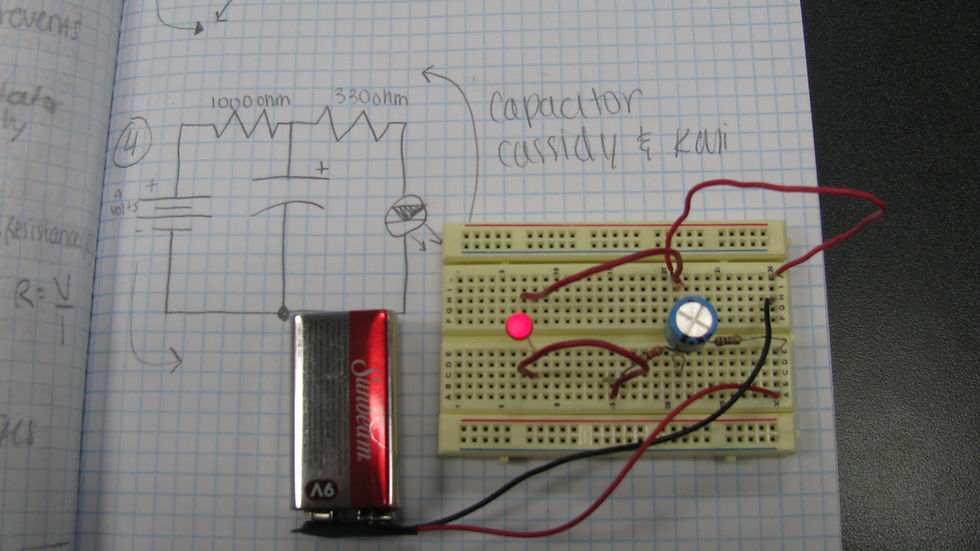top of pageEngineering Knowledge: Engineering Sciences## Engineering Performance Matrix

Circuit Theory is the collection of scientific knowledge used to describe the flow of electrical energy through an electrical circuit. This concept is important to Engineering Literacy as it enables an engineering professional to mathematically represent and verify how electrical components relate to one another in order to design and develop electrical circuits to perform specific tasks appropriately.

## Performance Goal for High School Learners

I can, when appropriate, draw upon the knowledge of Circuit Theory content, such as (a) series and parallel circuits, (b) Ohm’s Laws, (c) Kirchoff’s Laws, (d) resistance, capacitance and inductance, (e) wave forms, (f) signals, and (g) current, voltage, charge, energy, power, and work, to design, and mathematically justify, an electrical circuit to solve problems in a manner that is analytical, predictive, repeatable, and practical.

## SERIES & PARALLEL CIRCUITS

I can identify structural differences between series and parallel circuits.

## OHM’S LAWS

I can describe the relationship between voltage, currents, and resistance, using Ohm’s laws.

I can explain resistance, capacitance, and inductance relationships between series and parallel circuits.

I can calculate voltage, current, and resistance in different types of circuits with Ohm’s laws.

I can determine and justify which configuration is more appropriate for my design.

I can apply Ohm’s laws to analyze imposed criteria and constraints and make a decision in designing an electrical circuit.

## KIRCHHOFF’S LAWS

I can describe the relationship between voltage, currents, and resistance, using Kirchhoff’s laws.

I can calculate voltage, current, and resistance in different types of circuits with Kirchhoff’s laws. I understand the shortcut equations (nodal and loop) of Kirchoff’s Laws.

I can apply Kirchhoff’s laws to analyze imposed criteria and constraints and make a decision in designing an electrical circuit.

## RESISTANCE, CAPACITANCE, & INDUCTANCE

I can distinguish between resistance, capacitance, and inductance and describe their relationship.

I can calculate impedance, capacitance, and inductance, using equations illustrating their mathematical relationships.

I can infer criteria and constraints related to the use of impedances, capacitances, and inductances to solve a design problem.

bottom of page